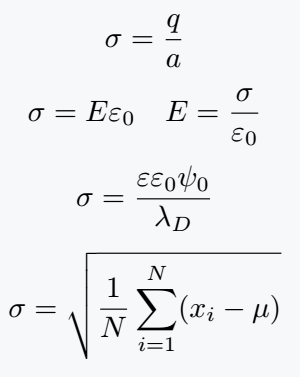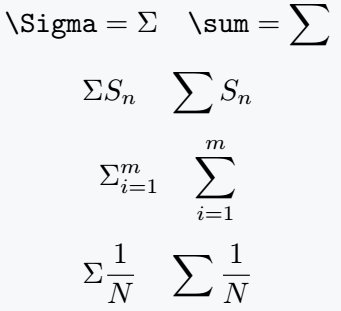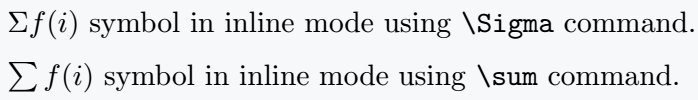# How to write Sigma(∑,σ) symbol in LaTeX?

In this content, we will discuss the Greek letter sigma. The Big Sigma symbol plays a major role in summation.

However, \Sigma command is not applicable for Big Sigma in terms of summation. There are usage errors for this which are discussed in detail below.

## Use small sigma symbol in latex

In latex, there is a \sigma command for the sigma symbol. In different cases, subscripts and superscripts are used with this symbol as you know.

Of course, the following output shows the different uses of the symbol.

\documentclass{article}
\begin{document}
$\sigma =\frac{q}{a}$
$\sigma =E\varepsilon_{0} \quad E=\frac{\sigma}{\varepsilon_{0}}$
$\sigma ={\frac{\varepsilon \varepsilon_{0}\psi_{0}}{\lambda_{D}}}$
$\sigma =\sqrt{\frac{1}{N}\sum\limits_{i=1}^N (x_i - \mu)}$
\end{document}

Output :## \Sigma and \Sum command

The following code shows the use of \sum and \Sigma commands. If you look closely, you will see a huge difference in the use of both commands.

\documentclass{article}
\begin{document}
$\verb|\Sigma|= \Sigma \quad \verb|\sum| = \sum$
$\Sigma S_n \quad \sum S_n$
$\Sigma_{i=1}^m \quad \sum_{i=1}^m$
$\Sigma \frac{1}{N} \quad \sum \frac{1}{N}$
\end{document}

Output :When using sigma commands, never match mathematical expressions. In case of inline mode, this symbol is used without limit.

In that case, also the difference between the two commands can be noticed.

\documentclass{article}
\begin{document}
$\Sigma f(i)$ symbol in inline mode using $\verb|\Sigma|$ command.\\ [6pt]
$\sum f(i)$ symbol in inline mode using $\verb|\sum|$ command.
\end{document}

Output :## Accurate limit position with sigma symbol

For the limit, you will need to use the subscript and superscript which you saw above.

But, with this symbol, you will face different problems with the limit.#### Md Jidan Mondal

LaTeX expert with over 10 years of experience in document preparation and typesetting. Specializes in creating professional documents, reports, and presentations using LaTeX.